# G0371033037 .pdf

### File information

Original filename: G0371033037.pdf
Author: hp

This PDF 1.5 document has been generated by MicrosoftÂ® Office Word 2007, and has been sent on pdf-archive.com on 13/11/2013 at 11:29, from IP address 182.74.x.x. The current document download page has been viewed 888 times.
File size: 236 KB (5 pages).
Privacy: public file

G0371033037.pdf (PDF, 236 KB)

### Document preview

International Journal of Computational Engineering Research||Vol, 03||Issue, 7||

Outage Analysis of Cooperation over Wireless Network
Sohrab Alam1, Sindhu Hak Gupta2
1,

2,

Department of Electronics &amp; Communication Engineering,
A.S.E.T, Amity School of Engineering &amp; Technology, Noida, Uttar Pradesh, India.

ABSTRACT
In this paper, we analyze the performance of cooperative communication system for independent
and flat Nakagami-m fading channel parameters by using amplify and forward cooperative protocol. We
have estimated the outage probability, channel capacity and ergodic capacity of the system. We further
simulate the equations of these parameters by plotting them for verification.

INDEX TERMS:

Diversity technique; cooperative communication; cooperative communication
protocols; Nakagami-m fading; ergodic capacity; channel capacity; outage probability.

I.

INTRODUCTION

An efficient technique of cooperation among the users having single antenna is required way to achieve
the transmit diversity in such a system which is unable to support multiple antennas either at the
transmitter/receiver side or on both sides due to size, cost, power, complexity of hardware , . So, the
virtual array of antennas is formed by cooperation in which the source broadcasts the multiple copies of the
signal toward the relays and destination with same power. Relays also forward the received copies of the same
signal toward the destination as shown in fig. 1. At the destination, we observe the resultant information after
maximum ratio combining (MRC) of all the multiple received copies of signal , , .

Fig.1 Cooperative communication
There are different types of cooperative communication protocols , , -:
1. Amplify and forward,
2. Detect and forward, and
3. Coded cooperation.
4.
In this paper, we use amplify and forward cooperative protocol strategy in which relays amplify the received
signals and retransmit them toward the destination as shown in figure 2.

Fig. 2. Amplify and Forward
Here, we have considered Nakagami-m fading channels to be independent and flat with parameter m.
www.ijceronline.com

||July ||2013||

Page 33

Outage Analysis Of Cooperation Over...
II.

SYSTEM MODEL

We have considered a system, which has a source (S), multiple relays i.e. R1, R2,R3, …Rn and the
destination (D) as shown in fig. 3.

Fig.3. Cooperative communication network
The channel coefficient between source and destination is flat Nakagami-m fading coefficient denoted by f. The
channel coefficient between source and ith relay is hi and between ith relay and destination is gi. These are the
flat and independent Nakagami-m fading channels.
Here, i= 1, 2, 3, 4,…….,n. The signals are transmitted in two phases.
In first phase, source transmits the signal’s one copy directly to the destination and multiple copies of the same
signal toward the relays with the same power (i.e. Ps). In second phase, all the relays Ri, where i= 1, 2, 3,
4,…….,n, transmit the amplified versions of received signal copies toward the destination with power PRi.
Where i= 1, 2, 3, 4,…….,n for n number of relays. Hence the total transmitted power toward the destination is
as
(1)
We also know that the ratio of transmitted power from source to destination to the total transmitted power
toward the destination is called the power distribution ratio i.e.
(2)
lies between 0 and 1. The power transmitted by each relay is equal to

.

Now, the instantaneous received power over source to destination link is expressed as

(4)

And the

instantaneous received power over ith relay to destination link is expressed as

By using maximum ratio combining (MRC), different independently faded versions are combined at the
destination and total received power at the destination is as

The maximum rate of transmission of information per second is the channel capacity per second which analyzes
the performance of the system. If the received power is so high due to which noise power is negligible, then
channel capacity by Shannon’s formula is as:

www.ijceronline.com

||July ||2013||

Page 34

Outage Analysis Of Cooperation Over...
After putting the value of

from equation (6) in equation (7), we get

(8)
For Nakagami-m distribution, Gamma distributed probability density function (PDF) is expressed as

After putting the value of

Here,

from equation (6) in equation (9), we get PDF as:

is the gamma function, parameter m is the shape factor and

The ergodic capacity analyzes the performance of the system which is the ensamble average of the information
rate over the distribution of the elements of the channel which is denoted as
or
=

(12)

III.

PERFORMANCE ANALYSIS

To analyse the performance of cooperative communication wireless system model, the outage probability
of the system also plays an important role in our study.
(a) Outage probability
To analyze the outage behaviour of cooperative communication wireless network, we select a threshold power
level ( ) in such a manner that if the total received power is below this level, then the system is said to be in
outage for this particular channel and the probability of the system in outage condition is called the outage
probability ,

After putting the value of

Where,

(13)
from eq. (10) in eq.(13), we get

(14)
is selected threshold power level , m is Nakagami-m parameter,

is total power received at the destination as mentioned earlier in

eq.(6).

www.ijceronline.com

||July ||2013||

Page 35

Outage Analysis Of Cooperation Over...
IV.

SIMULATION RESULTS

In this section, we have shown our results of outage probability, channel capacity and ergodic capacity
and total received power versus total average power at the destination. We get the simulated results using
MATLAB software.

Fig. 4. Outage probability V/S average received power at destination
Fig.4. shows the outage performance at different values of total average power. This curve shows that the outage
probability reduces fast as the value of total average power increases causing improvement in the performance
of the system. For a particular value of total average power at the destination node, the channel capacity and
ergodic capacity increase as shown in fig.5 and fig.6 respectively.

Fig.5. Channel capacity V/S average received power at destination
In this section, we have considered threshold power level

= 5 dB, Nakagami-m parameter (shape factor) m=2,

20 dB.

Fig. 6. Ergodic capacity V/S average power received at the destination
As the average value of received power (
) increases, the value of probability density function of Nakagamim fading distribution decreases resulting in the proper operation of the system due to lower outage probability
that is the probability of the system to be in outage state in which state, system does not work properly.

V.

CONCLUSION

From the simulation results, we can observe that system parameters such as channel capacity, ergodic
capacity increase and outage probability decreases as the value of average received power increases and it
shows that the system becomes more reliable and efficient.
www.ijceronline.com

||July ||2013||

Page 36

Outage Analysis Of Cooperation Over...
REFERENCES









A. Sendonaris, E. Erkip, and B. Aazhang, “User cooperation diversity-Part I: System description”, IEEE Trans. Commun., vol.
51, No. 11, pp. 1927-1938, Nov. 2003.
A. Sendonaris, E. Erkip, and B. Aazhang, “User Cooperation Diversity-Part II: Implementation aspects and performance
analysis”, IEEE Trans. Comm., vol. 51, no. 11, pp. 1939-1948, Nov. 2003.
J. N. Laneman, D. N. C. Tse, and G. W. Wornell, “Cooperative diversity in wireless networks efficient protocols and outage
behaviour”, IEEE Trans. Inf. Theory, vol. 50, no. 12, pp. 3062–3080, 2004.
J. N. Laneman and G. Wornell, “Energy-efficient antenna sharing and relaying for wireless networks”, in Proc. Wireless
Commun. Networking Conf. 2000, vol. 1, pp. 3062–3080.
J. N. Laneman and G. Wornell, “Distributed space-time coded protocols for exploiting cooperative diversity in wireless
networks”, in Proc. Glob. Telecomm. Conf. 2002, vol. 1, pp. 77–81.
Ankur Bansal and Parul Garg, “Performance Analysis of Coded Cooperation Under Nakagami-m Fading Channels”, in Proc.
IEEE Int. Conf. on Comm. (ICC), Cape Town, South Africa, May 2010.
J. N. Laneman, G. W. Wornell, D. N. C. Tse,”An efficient protocol for realizing cooperative diversity in wireless networks”, in
proc. IEEE Int. Symp. Inform. Theory, Washington, DC, pp. 294, Jun. 2001.
T. E. Hunter and A. Nostratinia, “Cooperation diversity through coding”, in Proc. IEEE Int. Symp. Inform. Theory, Laussane,
Switzerland, pp. 220, Jun. 2002

www.ijceronline.com

||July ||2013||

Page 37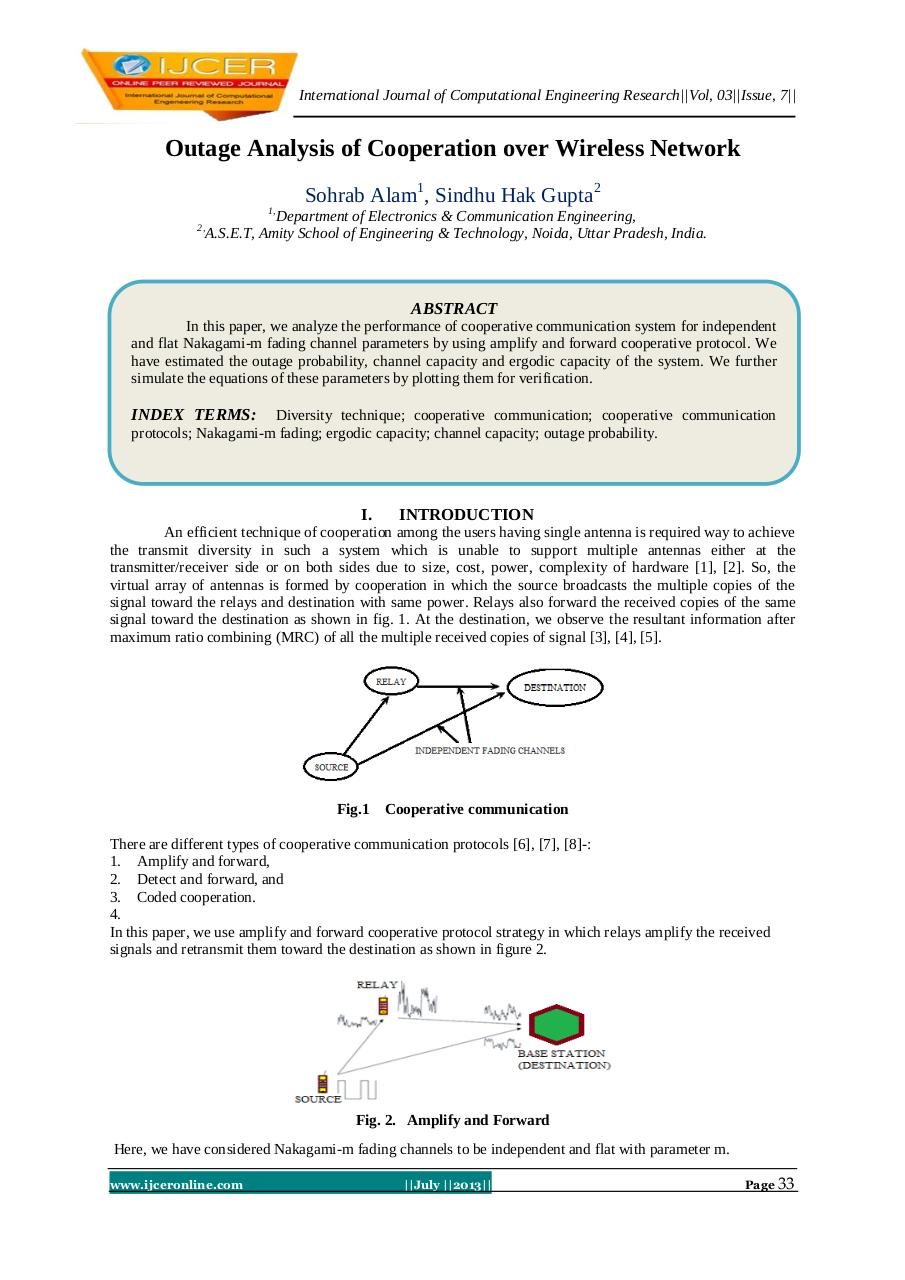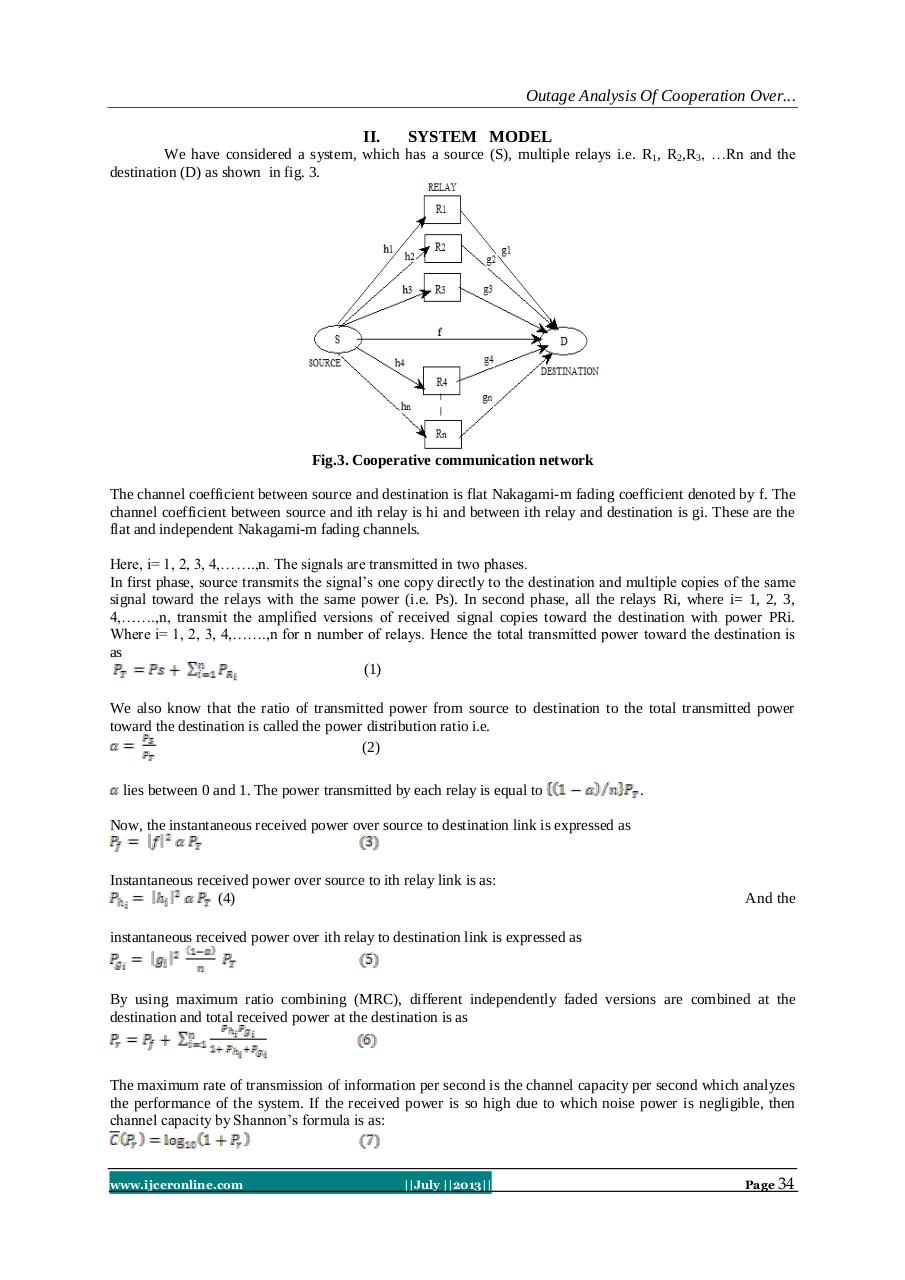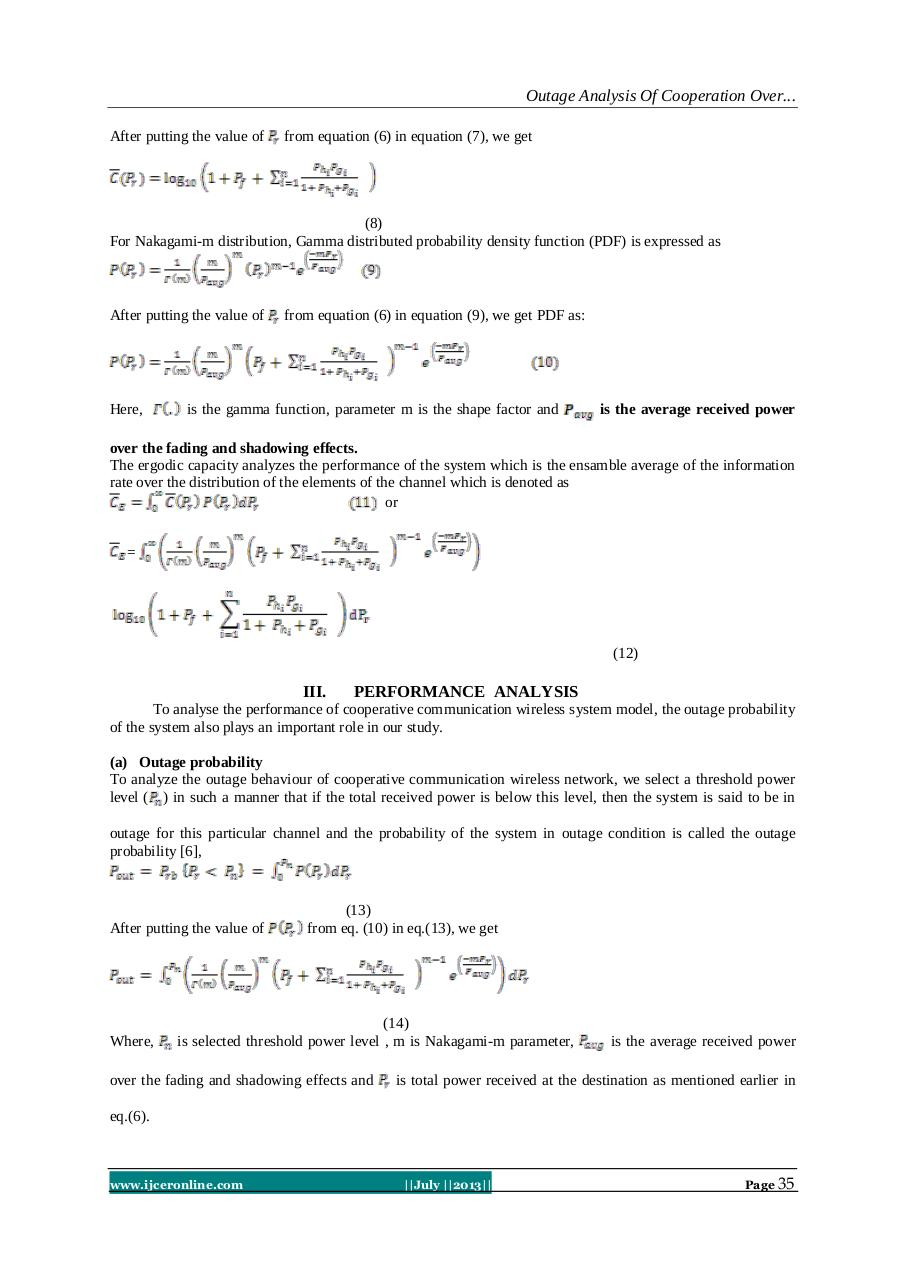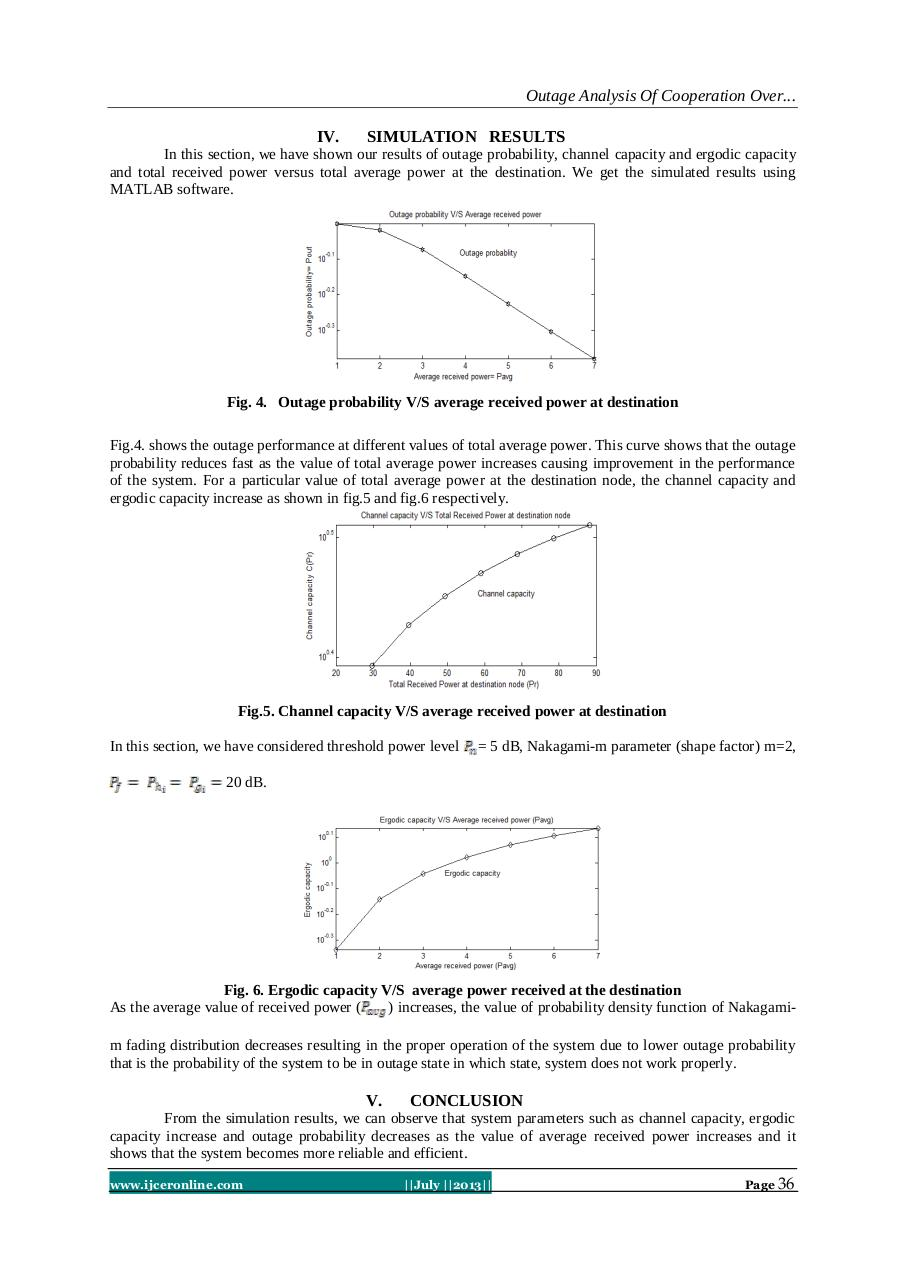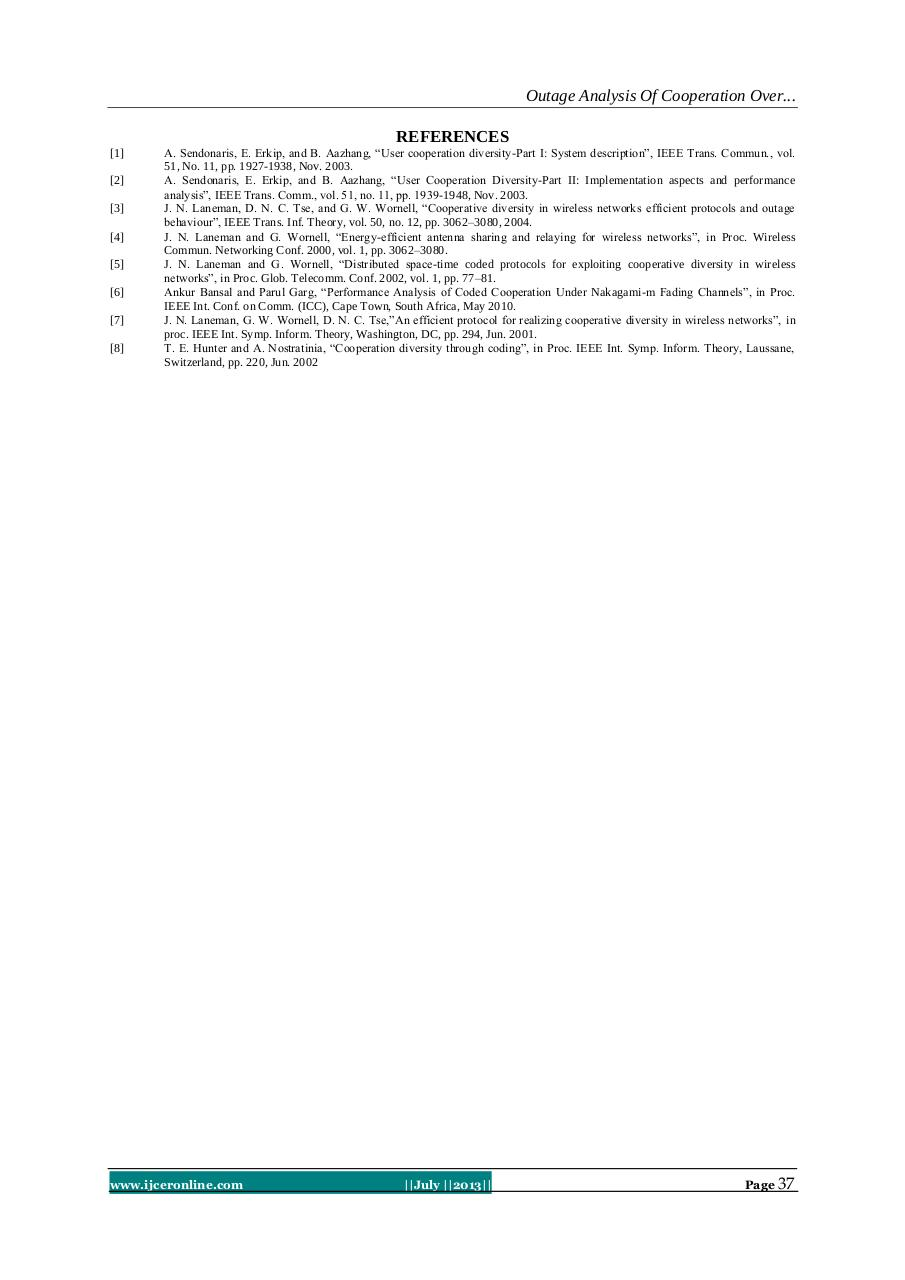#### HTML Code

Copy the following HTML code to share your document on a Website or Blog

#### QR Code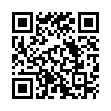### Related keywords